Courses

# Mirror And Water Images

## 20 Questions MCQ Test Science Olympiad Class 4 | Mirror And Water Images

Description
This mock test of Mirror And Water Images for Class 4 helps you for every Class 4 entrance exam. This contains 20 Multiple Choice Questions for Class 4 Mirror And Water Images (mcq) to study with solutions a complete question bank. The solved questions answers in this Mirror And Water Images quiz give you a good mix of easy questions and tough questions. Class 4 students definitely take this Mirror And Water Images exercise for a better result in the exam. You can find other Mirror And Water Images extra questions, long questions & short questions for Class 4 on EduRev as well by searching above.
QUESTION: 1

Solution:
QUESTION: 2

Solution:
QUESTION: 3

### In each of the following questions you are given a combination of letters and/or numbers followed by four alternatives (1), (2), (3) and (4). Choose the alternative which closely resembles the mirror image of the given combination.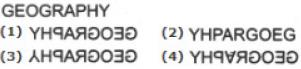Solution:
QUESTION: 4

In each of the following questions you are given a combination of letters and/or numbers followed by four alternatives (1), (2), (3) and (4). Choose the alternative which closely resembles the mirror image of the given combination.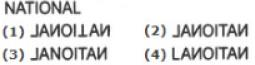Solution:
QUESTION: 5

In each of the following questions you are given a combination of letters and/or numbers followed by four alternatives (1), (2), (3) and (4). Choose the alternative which closely resembles the mirror image of the given combination.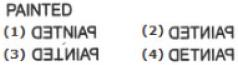Solution:
QUESTION: 6

Choose the correct mirror image of the given figure (X) from amongst the four alternatives.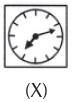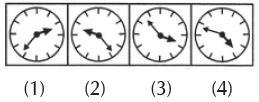Solution:
QUESTION: 7

Choose the correct mirror image of the given figure (X) from amongst the four alternatives.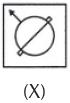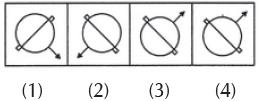Solution:
QUESTION: 8

Choose the correct mirror image of the given figure (X) from amongst the four alternatives.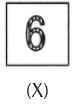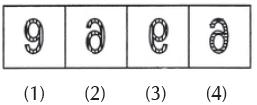Solution:
QUESTION: 9

Choose the correct mirror image of the given figure (X) from amongst the four alternatives.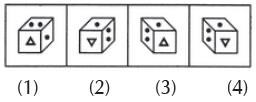Solution:
QUESTION: 10

Choose the correct mirror image of the given figure (X) from amongst the four alternatives.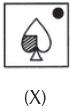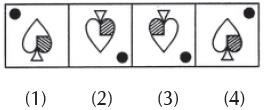Solution:
QUESTION: 11

Choose the correct mirror image of the given figure (X) from amongst the four alternatives.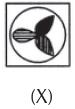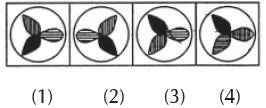Solution:
QUESTION: 12

Choose the correct mirror image of the given figure (X) from amongst the four alternatives.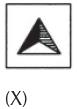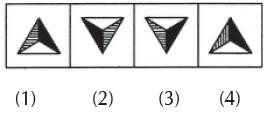Solution:
QUESTION: 13

Choose the correct water image of the given figure (X) from amongst the four alternatives.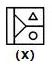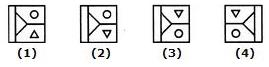Solution:
QUESTION: 14

Choose the correct water image of the given figure (X) from amongst the four alternatives.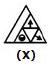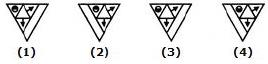Solution:
QUESTION: 15

Choose the correct water image of the given figure (X) from amongst the four alternatives.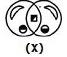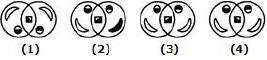Solution:
QUESTION: 16

Choose the correct water image of the given figure (X) from amongst the four alternatives.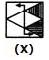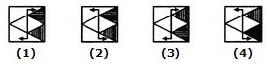Solution:
QUESTION: 17

Choose the correct water image of the given figure (X) from amongst the four alternatives.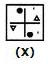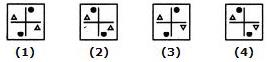Solution:
QUESTION: 18

Choose the correct mirror image of the given figure from amongst the four alternatives.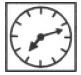Solution:
QUESTION: 19

Choose the alternative which closely resembles the mirror image of the given.
BRISK

Solution:
QUESTION: 20

Choose the alternative which closely resembles the mirror image of the given combination.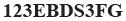Solution: